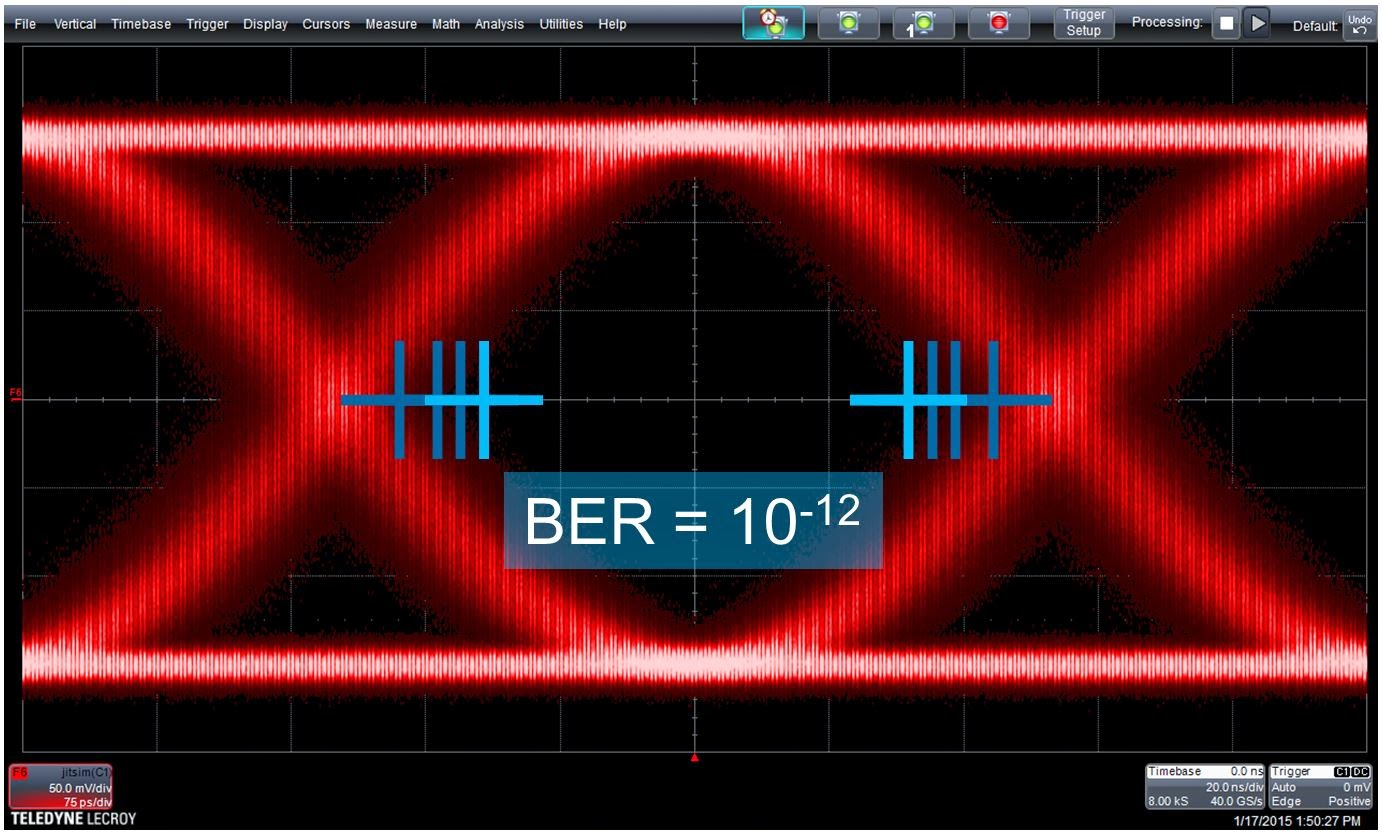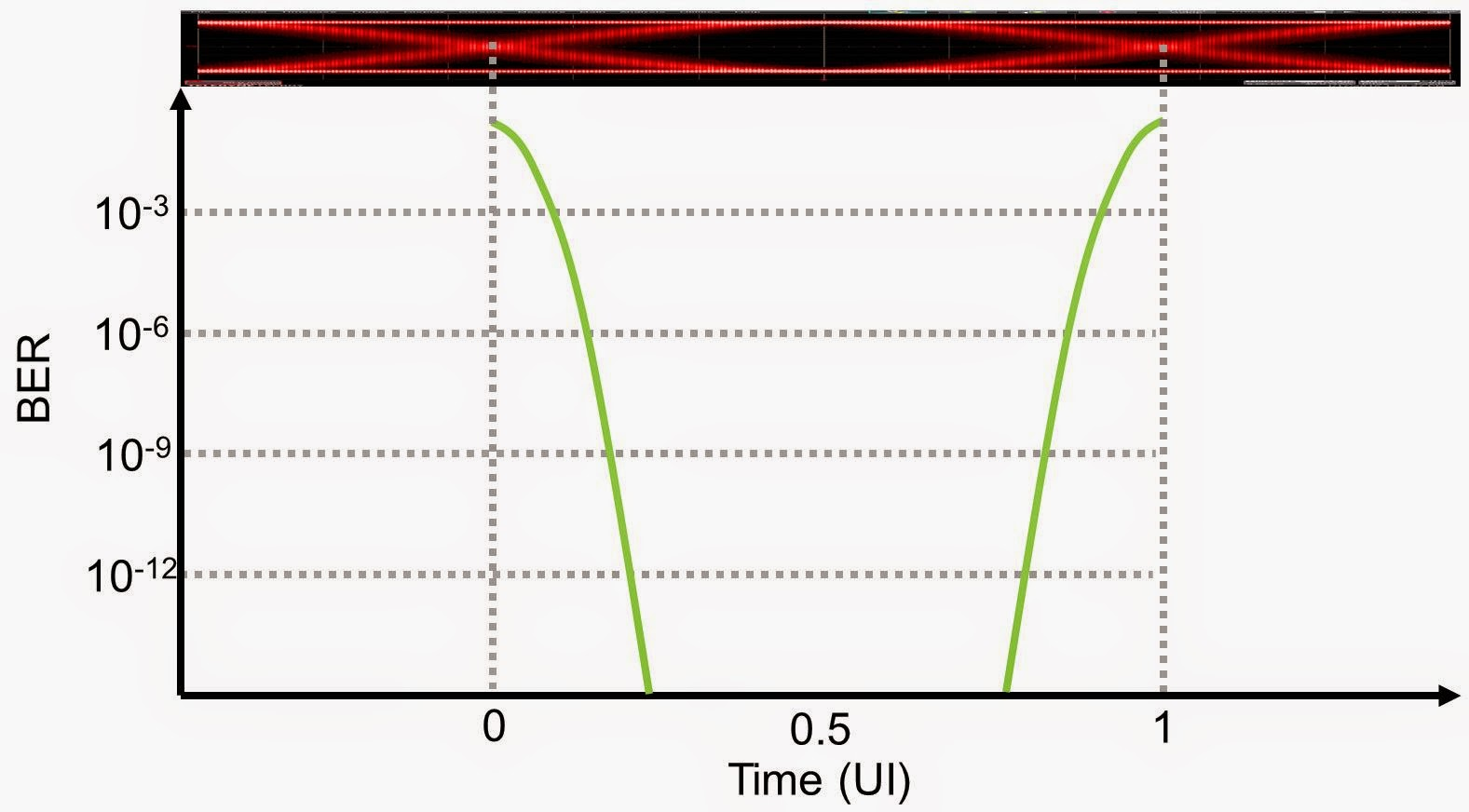## You need to test, we're here to help.

You need to test, we're here to help.

## 02 April 2015

### The History of Jitter (Part III)Figure 1: Latching a signal at the outermost of the blue hash marks results in a BER of 10-3, while latching it at the innermost hash marks yields a BER of 10-12
If you've been keeping track of our history of jitter, we left off in Part II in the late 1990s, by which time bit-error rates (BER) had become a predominant statistic for quantifying jitter. That was subsequently refined into thinking in terms of BER as a function of jitter.

Looking at BER as a function of jitter seen at a receiver depends on two things: how much jitter the signal has, and where the signal is being latched (Figure 1). Latching the signal close to the crossover point might yield a BER of 1 bit in 1000. Moving the latching point nearer to the middle of the eye gets the BER down to 10-12, or 1 bit in 1 million million bits.Figure 2: The classic "bathtub curve" relating a receiver's timing margins to BER based on the signal's jitter
If we could look at the BER at various points in the eye, which is the way a bit-error-rate tester (BERT) would characterize jitter, we could graph it as shown in Figure 2. The graph lets us look at BER in relation to our position in the unit interval. What we arrive at is the classic "bathtub curve" that is often associated with jitter measurements. The curve relates the receiver's timing margins to the BER based on the signal's jitter. It allows us to characterize the opening of the eye diagram, which, in terms of a BER value, will be determined by how many bits you look at.

The graph doesn't allow us to say declaratively that the eye is X picoseconds wide. But it does allow us to say that with a BER of, say, 10-3, that the eye is X picoseconds wide. Thus, we are able to measure the duration of the eye opening by measuring the BER at a given level at either side of the eye.

The inverse of the concept of "eye opening @ BER" discussed above is that of "total jitter (Tj) @ BER." The notion of how much jitter there is closing the eye is analogous to peak-to-peak jitter, but with a certain statistical significance. If we were to look at 1000 bits, what's the value of peak-to-peak jitter we should expect to see? If we were to look at 1 million bits, we would expect 10-9 or 10-12. So statistically speaking, we have an idea of peak-to-peak jitter but with a confidence factor.Figure 3: The Dual Dirac jitter model permits calculation of Tj @ BER at any arbitrary BER value
What the preceding paragraphs tell us is that it is rather difficult to measure BER values at different specific positions in the unit interval. It can be done but is time-consuming. So if we can't measure it, is there a way to use statistical data to model what will happen at any arbitrary BER value of our choosing?

If we gather a histogram of edge-crossing points, can we extrapolate out from that histogram to what the total jitter would be at a given BER? As it turns out, yes, we can, by fitting Gaussian functions to the tails of the distribution. Two values jump out that we need to fit: One is the sigma of the Gaussians, which corresponds to the random component of the jitter, and the other is the separation between the Gaussian mean values, which is the model's "view" of the deterministic jitter component. The deterministic components are what define the shape of the distribution between the tails.

What we're talking about is known as the Dual-Dirac model, which came to prominence in the Fibre Channel methodologies for jitter and signal quality dating to the late 1990s (Figure 3). If you can take your data and fit those two Gaussian functions to it, those two values (the Gaussian sigma and separation between Gaussian means) can be plugged into the equation in Figure 3 to extrapolate the total jitter expected at any given BER value. The Dual-Dirac model, at least theoretically, gave us a reliable and repeatable way to quantify jitter through statistical modeling.

Yet, having said all of that, tail fitting is a difficult problem. In fact, the same document that is the source for Figure 3 (Fibre Channel MJS, 1998) includes a footnote saying that "the most common technique for determining the best fit involves the human eyeball." Work was ongoing for development of a tail-fitting algorithm but the problem is that the tails of the histogram are, by definition, the area in which we have the least data to work with. Thus, it takes a large data set before the result converges.

Fortunately, changes would come as the 1990s ended and a new millennium began that would alter the jitter-measurement landscape considerably. We'll pick up the history of jitter in a forthcoming post.